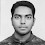## Atoms, Molecules and Ions

### Study Points

1. The law of conservation of mass states that there is no detectable loss or gain in total mass of the substances in a chemical reaction.

2. Law of Conservation of mass was proposed by the French chemist Antoine Lavoisier (1774)

3. The Law of constant proportion or definite proportions states that all pure specimens of the same compound contain the same elements combined together in the same proportion by weight.

4. Law of definite proportion was proposed by Louis Proust (1799).

5. An atom is the smallest particle of the element which can take part in chemical change and it can exist independently and retain all its chemical properties.

6. The smallest particle of matter made up of two or more atoms, which can exist in free state, is called a molecule.

7. Law of definite proportion does not hold good when the compound is obtained by using different isotopes of the combining elements .

8. Each atom of an element shows all the properties of the element.

9. The properties of a substance are the properties of its molecules.

10. A symbol is an abbreviation for the full name of an element.

11. The number of atoms contained in a molecule of a substance (element or compound) is called its atomicity.

12. The atomic mass unit (amu) is equal to one-twelfth (1/12) the mass of an atom of carbon-12.

13. The valency of an element is its capacity to combine with other elements.

14. Based upon atomicity molecules can be classified as mono-atomic, di-atomic and tri-atomic.

15. An ion is a charged particle formed by loss or gain loss or gain of electrons.

16. A chemical formula is a short method of representing chemical elements and compounds.

17. The empirical formula of a compound gives the simplest whole-number ratio of atoms of the elements in the molecule.

18. The molecular formula represents a chemical compound using symbols of atoms and shows the actual number of atoms of each element of the compound.

19. The mole (mol) is the amount of a substance that contains as many elementary entities as
there are atoms in exactly 12.00 grams of 12C

20. The atomic mass of an element expressed in grams is called gram atomic mass.

21. The Avogadro constant is named after the early nineteenth century Italian scientist Amedeo Avogadro.

22. 1 mole = 6.022 x 1023 particles

23. The relative atomic mass of an element is the mass of one atom of the element relative to one-twelfth (1/12) the mass of one carbon-12 atom.

24. The relative molecular mass of a substance is the mass of a molecule of the substance as compared to (1/12) the mass of one carbon-12 atom.

25. Gram molecular mass is the mass in grams of one mole of a molecular substance.1.who is the owner of this blog? this is awesome. i want to thank her/him

1.2.3.when we read this it is A PIECE OF CAKE to understand. thank you

4.this person is just awesome....now i m goin to use only this blog........

5.6.7.okkk!!! its great butttttt...one thing i want to say is that , they are too less..we study more than this..i knw these all points commonly please write some points that are more useful...thnx ...but overall its helpfull..

8.pls give some numericals also

1.Yeah...numericals are desperately needed.